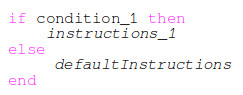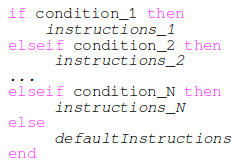# Scilab programming – IF ELSE conditional statements

• IF ELSE conditional statements in Scilab
• IF ELSEIF conditional statements in Scilab

When building complex algorithms for sure we’ll come across conditional statements. By conditional statement we understand the execution of a statement (instruction) only if a condition or a set of conditions is true.

Examples of conditional statements:

• if a person’s age is below 18 years, it’s forbidden to drive a car
• if the air temperature is above 30 °C, the weather is very hot

#### IF ELSE conditional statements

The general structure of an IF ELSE statement in Scilab is:A quite easy example is to define if it’s day or night function of the current hour. Let’s use the 24 hours format. If the current hour is between 7:00 and 19:00 we say it is day. If the current hour is NOT between 7:00 and 19:00 we say it’s not day (it is night).

The best way to practice this example is by using the Scilab script editor, SciNotes, in which we write the following Scilab instructions:

currentHour = 14;

if (currentHour > 7) & (currentHour < 20) then
outsideIs = 'DAY';
else
outsideIs = 'NOT DAY';
end

disp(currentHour)
disp(outsideIs)

The currentHour variable is our control variable. We check its value against the limit values for day. In the condition slot we check if two conditions are TRUE with a “&” (AND) operator. We do this because in order to say if its day we have to check that the current hour is between minimum (7:00) and maximum (20:00) hours.

Next we use the string variable outsideIs which can take two values DAY or NOT DAY depending on the current hour.

For the else statement we do not explicitly have a condition, the else statement is executed if the if condition is FALSE.

If we execute the above script we’ll see displayed in the Scilab console:

14.

DAY

This means that when the current hour is 14:00 o’clock, according to our conditions, outside is day. Now we’ll change the value of the currentHour variable to 22 and run the script againg. In the Scilab console we’ll see:

22.

NOT DAY

This means that when the time is 22:00 o’clock outside is not day, it is night.

#### IF ELSEIF conditional statements

If we have more than one condition, we need to use the IF ELSEIF statements.For understanding of the IF ELSEIF conditional statements we are going to use as example the definition of the sign function. The sign function outputs 1 if the argument is a positive number, -1 if the argument is negative and 0 if the argument is 0:

$\begin{equation*} \begin{split} \operatorname{sign}(x):={\begin{cases}-1&{\text{if }}x<0,\\0&{\text{if }}x=0,\\1&{\text{if }}x>0.\end{cases}} \end{split} \end{equation*}$

Scilab has it’s own built-in sign function called sign().

-->sign(-3)
ans =

- 1.

-->sign(4)
ans =

1.

-->sign(0)
ans =

0.

-->

In the image below you can see a logical diagram of the IF ELSEIF conditional statement for the sign() function:

Example: A normal, average work day starts at 8:00 o’clock and ends around 16:00 o’clock. Depending on the daily activities we can split the work hours as:

• 8:00 – 10:00 Project Meetings
• 10:00 – 12:00 Training
• 12:00 – 12:30 Lunch Break
• 12:30 – 16:00 Project Development
• 16:00 – 8:00 Free Time

Using ELSEIF statements design a script which displays in the Scilab console what activity is performed function on the current hour.

currentHour = 9;
if (currentHour > 8) & (currentHour <= 10) then
disp("Activity: Project Meetings")
elseif (currentHour > 10) & (currentHour <= 12) then
disp("Activity: Training")
elseif (currentHour > 12) & (currentHour <= 12.3) then
disp("Activity: Lunch break")
elseif (currentHour > 12.3) & (currentHour <= 16) then
disp("Activity: Project Development")
else
disp("Activity: Free Time!")
end

IF ELSE conditional statements are very common in any programming language. Do a couple of exercises to practice the syntax and you’ll be in a good position to use it where necessary.

For any questions, observations and queries regarding this article, use the comment form below.

Don’t forget to Like, Share and Subscribe!